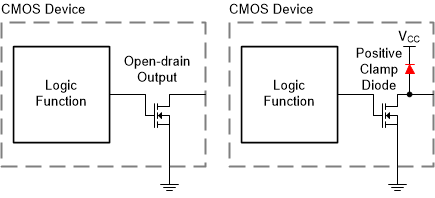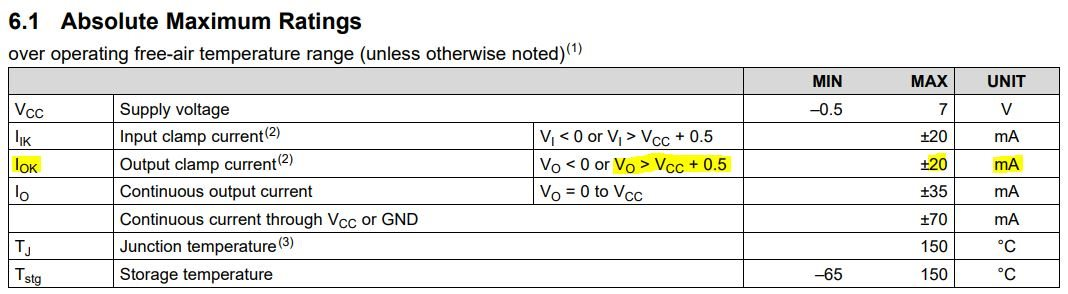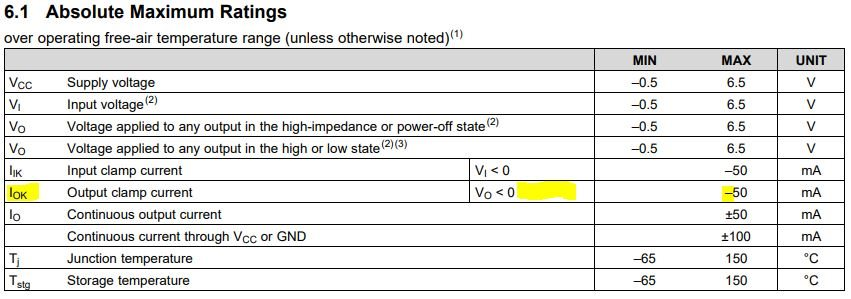If you have a related question, please click the "Ask a related question" button in the top right corner. The newly created question will be automatically linked to this question.

• Resolved

# [FAQ] Can you pull-up an open-drain output to a higher voltage than the device's supply (VCC) voltage?

FAQ: Logic and Voltage Translation > Output Parameters >> Current FAQ

This question is really about the output structure of the logic device.

There are primarily two types of open-drain output structures - one with a positive clamping diode, and one without, shown here:The positive clamp diode is shown in red.  If the output voltage is higher than Vcc, then the positive clamp diode will become forward biased and will allow current to flow through to Vcc.  This has two primary effects.  First, it can provide power to the Vcc rail (this is an example of "back-powering" a device).  Second, it's possible for the current through the clamp diode to become so large that it can permanently damage the device. This current limit is rated as "I_OK" in the datasheet, and is found in the absolute maximum ratings table.  Here's an example of a device's abs max table with an input clamp diodeNote that the output clamp current can be turned on two different ways - going into the part (+20mA) and out of the part (-20mA) and there is a condition of V_O < 0 or V_O > Vcc + 0.5V. These are two indicators that there is a positive clamp diode.  Here's an example abs max table for a device without a positive clamp diode:In this table, you can see that the positive current value and the condition of V_O > Vcc + 0.5V are both absent. This indicates that there is no positive clamp diode.

Another indicator is the maximum value on the output voltage. For a device with a clamp diode, the output voltage will be limited to Vcc or Vcc + 0.5V, however a device without the positive clamp diode will have a numerical value.

There is one more possibility for the output being a higher voltage than the supply, which is the case where the supply is off (Vcc = 0V) and the output is operating at some voltage higher than 0V.  This case is covered partially by the above information, but also has a special feature associated with it called "Ioff - Partial Power Down Protection".

If a device is rated for operation with Vcc = 0V, the Electrical Characteristics table will include the value of Ioff, which is the leakage current into any input or output pin of the device when the supply is forced to 0V. Note that this spec is _only_ valid under the case that the supply is at 0V, and not just if the supply is disconnected (floating) or off (unknown voltage). Some supplies can still provide enough voltage to operate a device even though they are disabled -- for example, many boost converter circuits have a direct connection from the input voltage to the output through only a diode.

• FAQ